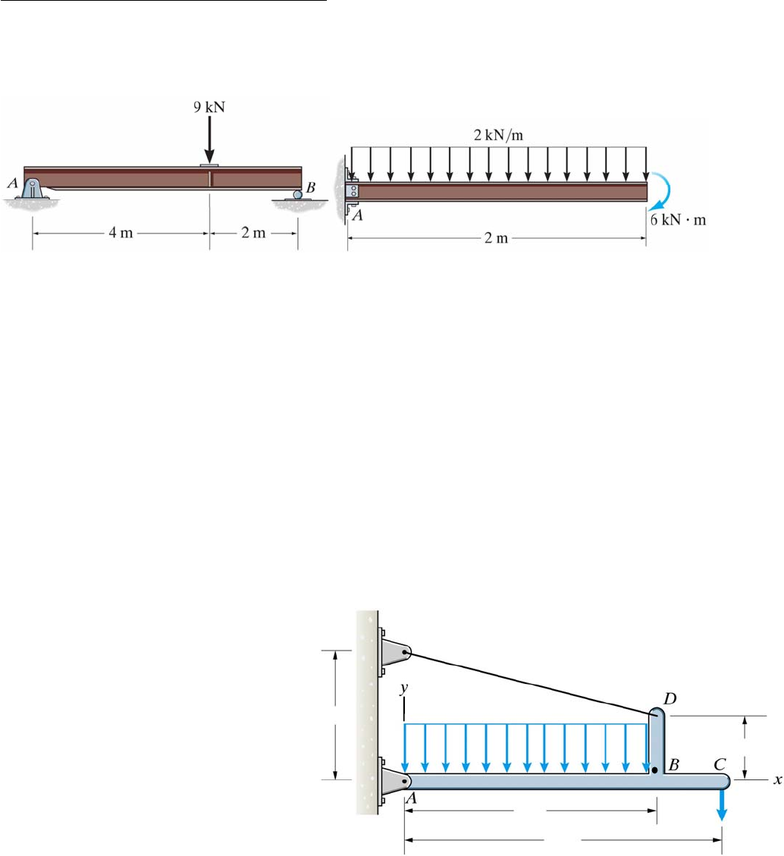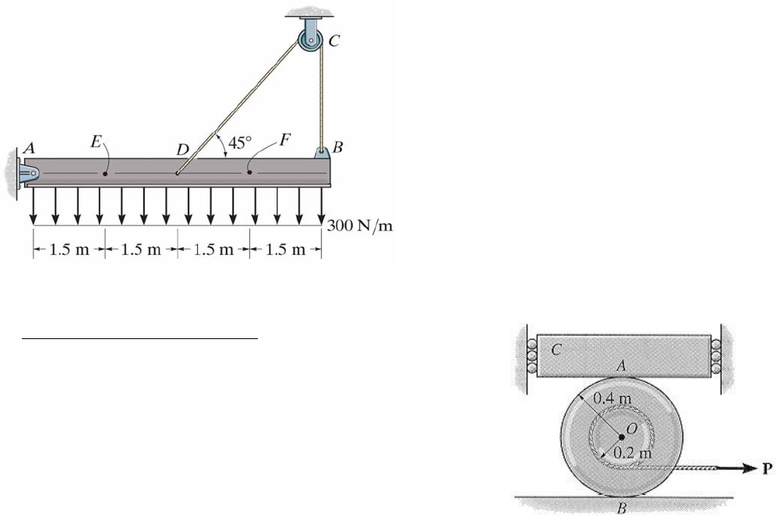# ENGG 202 Lecture Notes - Lecture 1: National Air Force Of Angola, Rolly Teranishi, A Roads In Zone 2 Of The Great Britain Numbering Scheme

74 views5 pagesENGG 202 Tutorial 13 April 9/10, 2013
Shear Force and Bending Moment Diagrams
1) Draw the shear and moment diagrams for the two beams shown below.
ANS: (a). Vleft = +3kN, constant up to point load then jumps to – 6 kN, Vright = -6 kN, M = 0 at ends, Mmax = 12 kN-
m at location of point load, linear variation (b) Vmax = 4 kN at A, linear up to VB=0, Mmax = -10 kN-m at A, parabolic
up to M = -6 kNm at B.
Solution Strategy: From FBD of each beam find reactions at A (and B for the first beam). (a)
Section the beam between A and the point load, and again between the point load and B. draw F,
V, and M, write Eqns of Equil to get equations for V and M at each section. Calculate V and M at
critical points (A,B,and location of point load). (b) Section the beam between A and the end of
the beam (section the distributed load as well). draw F, V, and M, write Eqns of Equil to get
equations for V and M at each section. Calculate V and M at critical points (each end of beam).
2) A roof structure is represented by the member ABCD and loading shown below. Neglecting
the weight of the member:
(a) Determine the magnitude and direction of the reactions at point A and the magnitude of the
force in cable DE.
(b) Determine the internal axial force, shear force, and bending moment at point B by
sectioning through BD and drawing the Free Body Diagram of segment BD.
(c) Draw the shear force and bending moment diagrams for beam ABC. Indicate the values for
shear force and bending moment at points A, B, and C.
ANS: a) TDE = 1587.2 N, Ax = 1540 N right, Ay
= 255 N up b) N = 385.1 N down, V = 1540 N
right, M = 1540 Nm CW c) SFD starts at +255 N
linear decrease to 215 N at 4 m, jump up to 600 N
at 4 m, constant to end of beam, BMD starts at 0,
parabolic (upside down) increase up to 940 Nm at
4 m, jump down to -600 Nm at 4 m, linear
increase to 0 at right end of beam. must show
calculations for the key values at ends of beam
and at 4 m.
Solution Strategy: a) draw FBD of bar
ABCD and solve for tension force and
reactions at A using eqns of equil. b) section horizontally through segment BD at B. draw the
FBD of the segment BD (tension force at D and internal forces/moment at B). use eqns of equil
to solve for the internal forces/moment. c) draw FBD of segment ABC (reactions at A,
distributed load, force at C, internal forces from b) act at B but are equal and opposite to those
computed in b)). SFD and BMD can be obtained either by section between A and B and again
2 m
4 m
5 m
1 m
600 N
10 N/m
E
Unlock document

This preview shows pages 1-2 of the document.
Unlock all 5 pages and 3 million more documents.ENGG 202 Tutorial 13 April 9/10, 2013
between B and C, then using equations of equil to get equations for V and M as a function of x.
alternatively, use the relationship between the diagrams: 1) for the SFD: at A, V = the reaction
Ay, then it decreases linearly at a slope of -10 N/m up to point B. The axial force from part b)
causes a jump upward = to that force (jumps to 600 N). Then constant to the end of the beam at
600 N. 2) for the BMD: starts at M=0 at point A. moment diagram is parabolic up to point B
(concave downward). The moment at B can be computed from the area under the shear diagram
between A and B. At B there is a jump down of 1540 Nm to -600 Nm due to the internal moment
at B found in part b). there is a linear increase (slope = shear = +600) over the last m back up to
M = 0 at point C.
3) Determine the internal axial force, shear
force and bending moment at points E and F
of the beam shown.
ANS: VF = -215 N, NF = 0, MF = 660 N-m, NE = 470
N, VE = 215 N, ME = 660 N-m
Solution Strategy: From FBD of AEDB
find reactions at A and tension in the cable.
Section member at E (section distributed load
too, and consider left section). Draw N, V,
and M at point E. Write Eqns of Equil and
solve. Repeat for point F (consider right
section).
Friction and Centroid Problems
4) Block C has a mass of 50 kg and is confined between two
walls by smooth rollers. If the block rests on top of the 40 kg
spool, determine the required coefficients of static friction at
A and B so that the spool slides (doesn’t roll) at A and B when
the magnitude of the applied force is P = 300 N.
ANS: at A: 0.153, at B: 0.255
Soln Strategy: this is an impending motion problem (“required coefficients”) draw the FBD of
the spool. The normal force at A is equal to the weight of the block (we know this from FBD of
the block). just before the spool slides, the friction force at each of A and B reaches the maximum
friction force. Since the spool slides and doesn’t roll both friction forces are to the left. Use Eqns
of Equil to solve for the friction forces at A and B and the normal force at B. the friction coeff at
A is FA/NA and likewise for B.
Unlock document

This preview shows pages 1-2 of the document.
Unlock all 5 pages and 3 million more documents.